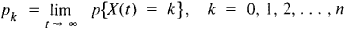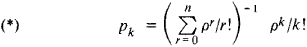queuing theory

(redirected from Waiting Line Theory)
Also found in: Dictionary, Medical, Financial.
Related to Waiting Line Theory: Queuing theory

queuing theory

The study of how systems with limited resources distribute those resources to elements waiting in line, and how those elements respond. Examples include data traversing computer networks, phone calls traveling over voice networks and the distribution of cars on highways. Queuing theory is a complex area of engineering that is closely related to traffic engineering. See queuing and traffic engineering methods.

Queuing Theory

a mathematical discipline that studies systems intended for servicing a random flow of requests (the moments at which the requests appear as well as the time for servicing them may be random). A typical field of application of queuing theory is to automatic telephone exchanges to which requests for service—namely, the calls of the subscribers—arrive in a random fashion and where service consists in connecting subscribers to other subscribers and in maintaining the connection for the duration of the call. The purpose of methods developed in queuing theory is to organize service reasonably so that a given quality is ensured. Queuing theory from this standpoint can be considered as part of operations research.

Queuing theory widely uses the apparatus of probability theory and, to a lesser degree, mathematical statistics. Problems in queuing theory formulated mathematically usually reduce to the study of a special type of random process. Proceeding from given probabilistic characteristics of the incoming flow of calls and the service period and taking into account the organization of the system of service (presence of failures or of queues), queuing theory determines the corresponding characteristics of the quality of service (failure probability, mean time for service, mean down-time of communication lines). This determination is possible using analytic methods in a number of simpler cases, although more complex cases require the simulation of the corresponding random processes by means of a Monte Carlo method.

Example. Let us assume that an automatic communication system has n identical channels available to subscribers. Calls come in at random moments of time. If all the n channels of the communication system are busy when a new call arrives, that call will fail and be lost. If they are not all busy, a conversation will immediately begin on one of the free channels and in general will continue for a random period of time.

A measure of the operational efficiency of such a communication system is the fraction of calls that fail, that is, the limit p as T —. ∞ (if it exists) of the ratio vT/NT of the number VT of calls lost over a period of time of length T to the total number NT of incoming calls. This limit can be called the failure rate.

Another and no less essential measure of the operational efficiency of a communication system is the relative time during which it is busy, that is, the limit p* as T — ∞ (if it exists) of the ratio TT/ T, where TT is the total time during which all n channels of a communication line are simultaneously busy during a time period of length T. This limit may be called the busy rate. Let X(t) be the number of channels that are busy at time t. We can then show that (1) if the moments at which the calls come in form a time-homogeneous Poisson process of events and (2) if the lengths of conversations of successive subscribers are identically distributed, random variables, independent of one another and of the time at which the conversation begins, then the random process X(t), where t ≥ 0, possesses an ergodic distribution, that is, there exist [independently of the initial distribution X(0] the limitsand furthermorewhere p is the product of the arrival rate of incoming calls and the mean length of a conversation of an individual subscriber. Moreover, in this case, p = p* and their common value is equal to pn. The formulas (*) are used for calculating the minimum number of communication line channels that guarantee a given failure rate. These formulas are called Erlang formulas. It should be noted that if condition (1) is not satisfied, then the equality p = p* may not hold.

Queuing theory grew out of the interest the Danish engineer K. Erlang had in mathematical problems connected with organizing telephone networks. Erlang’s first published works appeared in the 1920’s. The theory was further developed in the 1950’s by C. Palm (Sweden), F. Pollaczek (France), and A. Ia. Khinchin (USSR). Their studies have been continued by the Soviet mathematician B. V. Gnedenko and others. The development of queuing theory has been significantly stimulated by the expansion of its range of application. Although formally part of the theory of random processes, queuing theory has become an independent field of research with its own set of problems and methods of solution and has in turn stimulated the development of the theory of random processes.

REFERENCES

Khinchin, A. Ia. Raboty po matematicheskoi teorii massovogo obsluzhivaniia. Moscow, 1963.
Rozenberg, V. Ia., and A. I. Prokhorov. Chto takoe teoriia massovogo obsluzhivaniia. Moscow, 1965.
Gnedenko, B. V., and I. N. Kovalenko. Vvedenie v teoriiu massovogo obsluzhivaniia. Moscow, 1966.
Saaty, T. L. Elementy teorii massovogo obsluzhivaniia i ee prilozheniia. Moscow, 1971. (Translated from English.)
Borovkov, A. A. Veroiatnostnye protsessy v teorii massovogo obsluzhivaniia. Moscow, 1972.

O. V. VISKOV

Site: Follow: Share:
Open / Close